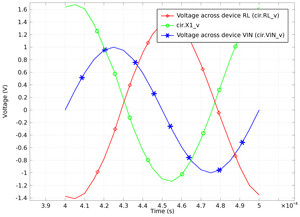# Application Gallery

## Inductor in an Amplifier Circuit

Application ID: 990

This model shows how to combine an electric circuit simulation with a finite element simulation. The finite element model is an inductor with a nonlinear magnetic core and 1000 turns, where the number of turns is modeled using a distributed current technique.

The circuit is imported into COMSOL Multiphysics as a SPICE netlist, which merges the inductor model and the circuit elements as ODEs.This model example illustrates applications of this type that would nominally be built using the following products: